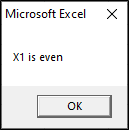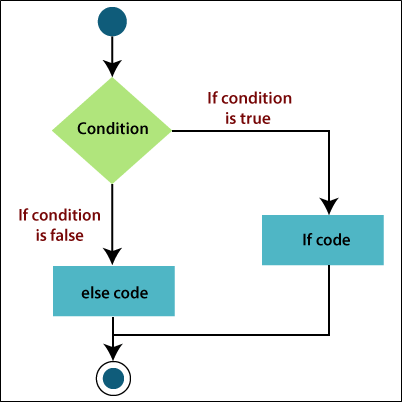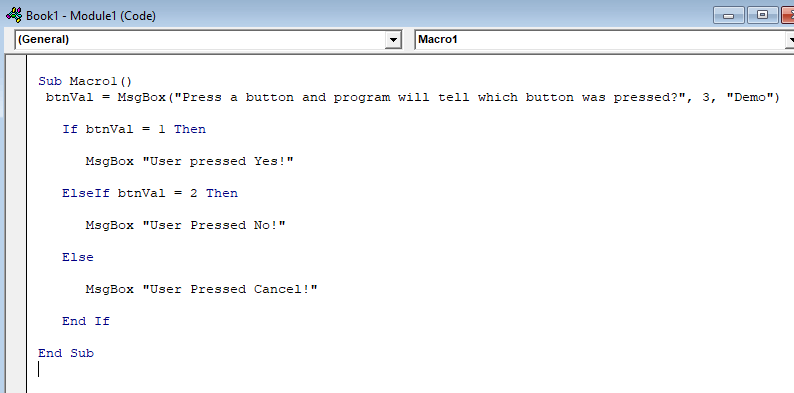# If Else If Statement

If Then, If Then Else and If Else If statements allows the programmers to control the execution flow of a script or one of its sections.

Below image is the general form of structure found in the most of the programming languages.## 1. If Then statement

An If Then statement contains a Boolean expression that allows one or more statements.

If the condition is True, the statements under If condition(s) are executed. If the condition is False, the statements after the If loop are executed.

Syntax

To run one statement when a condition is True, we can use single line syntax of the If statement.

For example:

And to run more than one line of code, than you can use the multiple-line syntax. This syntax includes the End If statement.

For example:

Flow DiagramExample

Let’s find the largest between the two numbers of Excel with the help of a function.

After that executes the above code, and you will get the output such as:

`x is greater than y   `

Here are some more examples of the If Then statements. This VBA code is checking whether the specified condition evaluates to True or False.

When the condition evaluates to True, you have only specified the steps to be completed. In this case, an MsgBox will be displayed on the screen as the result of the code.

And if the condition evaluates to False, the VBA code will do nothing, and it will skip the line instantly.

For example:In the above example, if the number contained in the cell of excel has the remainder zero when divided by 2, then excel displays a MsgBox that tells the number is even.## 2. If Then Else statement

An If statement contains a Boolean expression that allows one or more statements, if the condition is True, the statements under If condition is executed. If the condition is false, the statement under the Else condition is executed.

Syntax

According to the above syntax, you can perform different tasks according to the result of the condition.

For example:Now when you run your VBA code, you will get the output no matter what the numeric value in A1 is. You can see the output in the below screenshot of the above code.The If ThenElse statement executes one set of code if a specified condition evaluates true and another set of code evaluates false.

The If Then Else statement is a built-in function in excel, which is categorized as a logical function. It also can be used as a VBA function in excel. You can use this function in macro code that is entered through the Microsoft visual basic editor.

Flow DiagramExample

Let’s find the largest between the two numbers of Excel with the help of a function.

After that executes the above code, and you will get the output such as:

`y is greater than x  `

Let’s take one more example to clear the If Then Else statement.

For example, suppose you want to print the student result pass or fail in Excel VBA.

If the score is greater than or equal to 60, Excel VBA returns pass as a result, and else Excel VBA returns fail.

## 3. If ElseIf statement

An If statement allows one or more If Else statements that contain a Boolean expression. And it is then followed by a default Else statement that executes when all the statements become False.

Syntax

Not every condition can be reduced to a simple two statement. You also have more than two options in your code. In this situation, you can use ElseIf statement.

You can use more than one or two ElseIf parts, as many as you need as seen in the above syntax.

Flow DiagramExample

Let’s find the largest between the two numbers of Excel with the help of a function.

After that executes of the above code, and you will get the output such as:

`x and y are Equal  `

Let’s go for some more examples of If ElseIf statement.

For example, consider you have a three buttons dialogue box with Yes, No, and Cancel options.

If the user presses a button, you will get the button value and display a message to the user that tells to the user which button was pressed.

For that, the VBA Else If statement is as used as:You can see, how btnVal variable is evaluated in the If and ElseIf statements and then you will get the respective message to the user by another dialogue.After pressing any one button among all the three buttons or any key which you gives in the code, you will get the following output such as:Previous articleVBA Data Types
Next articleCircular reference in Excel